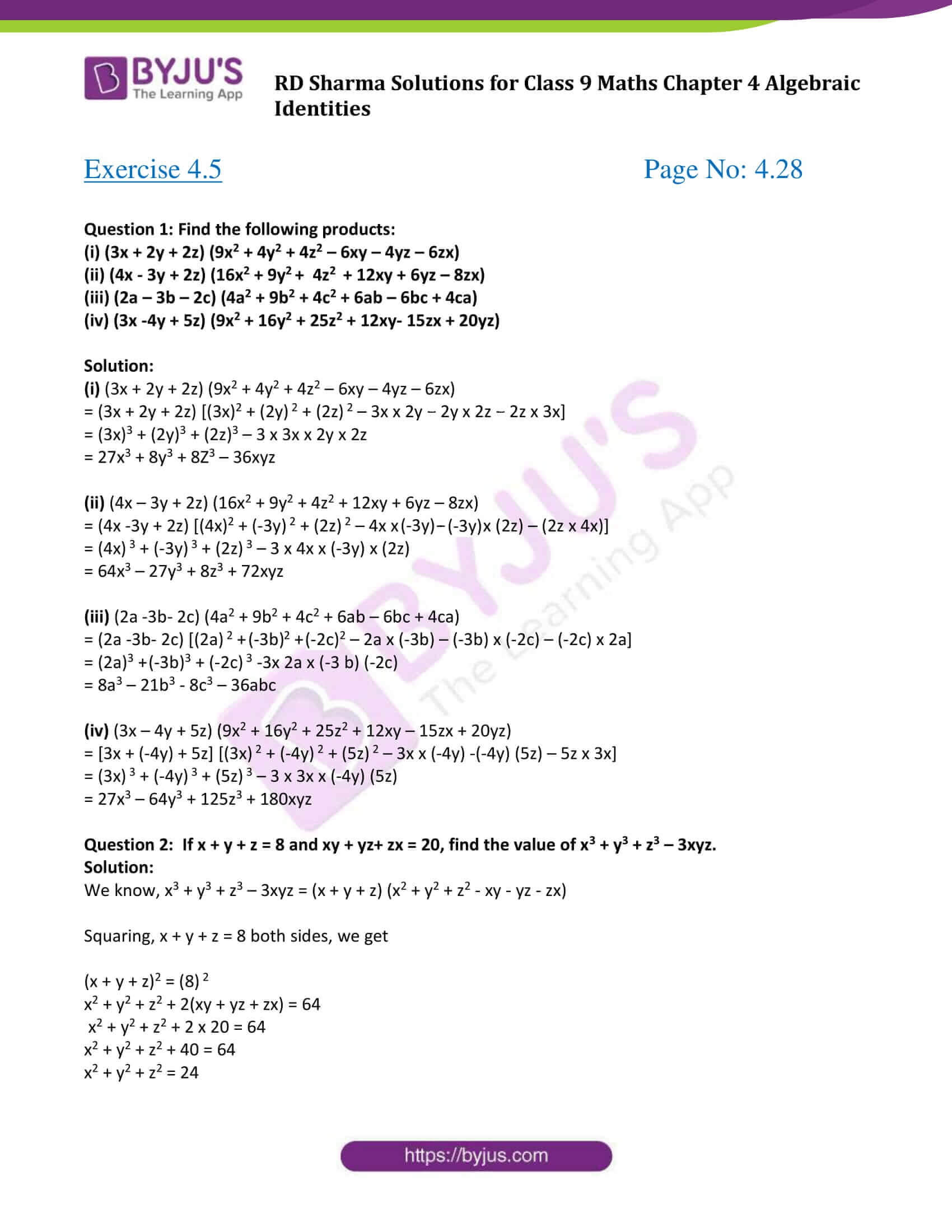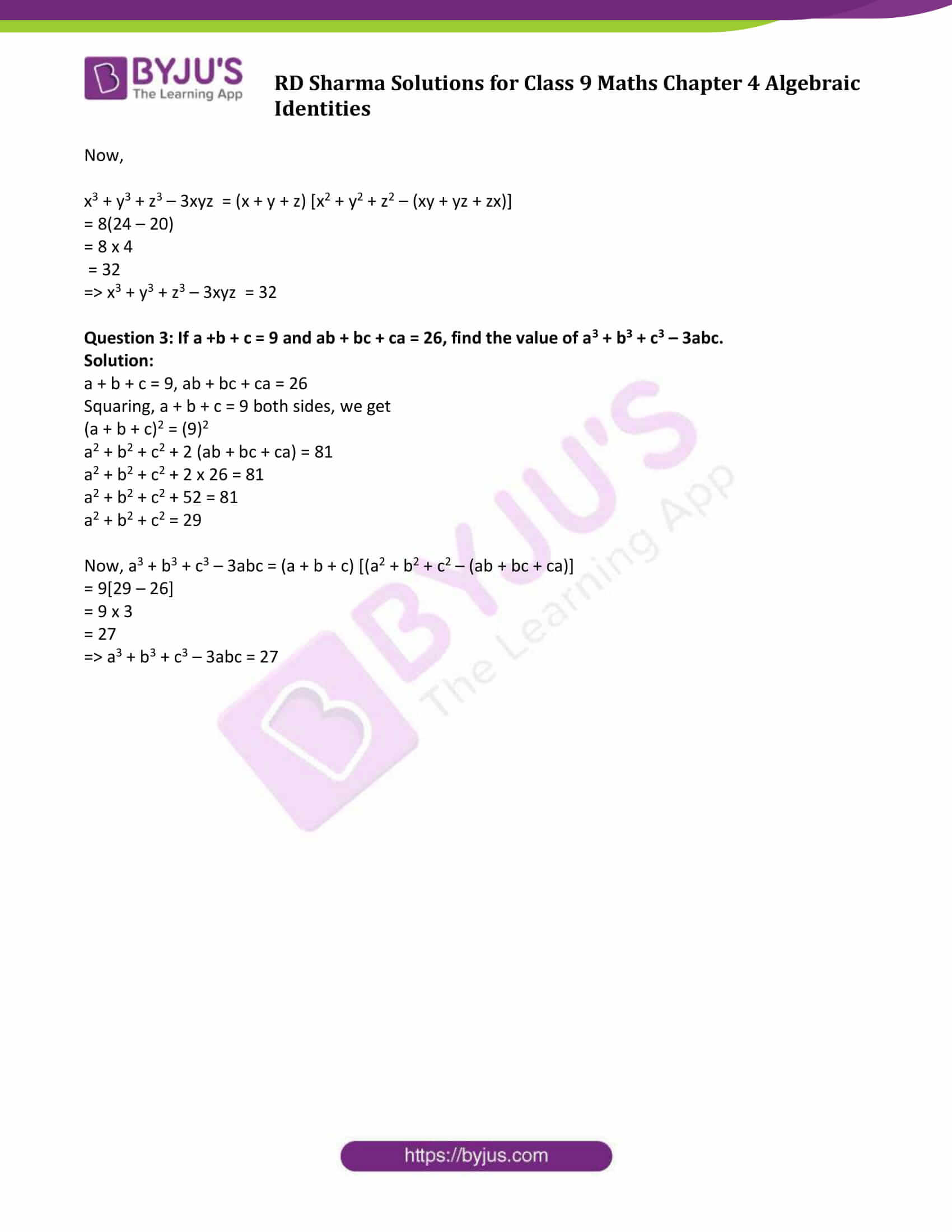# RD Sharma Solutions for Class 9 Maths Chapter 4 Algebraic Identities Exercise 4.5

RD Sharma Class 9 Maths Chapter 4 exercise 4.5 available here. This exercise is about the sum and difference of cubes identity and conditional identity. RD Sharma Class 9 Maths pdf contains detailed answers prepared by experts and will enable students to study effectively as well as to be ready to tackle any questions during the exams. Students are advised to practice this RD Sharma chapter in order to ace their examinations.

## Download PDF of RD Sharma Solutions for Class 9 Maths Chapter 4 Algebraic Identities Exercise 4.5### Access Answers to Maths RD Sharma Solutions for Class 9 Chapter 4 Algebraic Identities Exercise 4.5 Page number 4.28

#### Exercise 4.5 Page No: 4.28

Question 1: Find the following products:

(i) (3x + 2y + 2z) (9x2 + 4y2 + 4z2 – 6xy – 4yz – 6zx)

(ii) (4x – 3y + 2z) (16x2 + 9y2 + 4z2 + 12xy + 6yz – 8zx)

(iii) (2a – 3b – 2c) (4a2 + 9b2 + 4c2 + 6ab – 6bc + 4ca)

(iv) (3x -4y + 5z) (9x2 + 16y2 + 25z2 + 12xy- 15zx + 20yz)

Solution:

(i) (3x + 2y + 2z) (9x2 + 4y2 + 4z2 – 6xy – 4yz – 6zx)

= (3x + 2y + 2z) [(3x)2 + (2y) 2 + (2z) 2 – 3x x 2y – 2y x 2z – 2z x 3x]

= (3x)3 + (2y)3 + (2z)3 – 3 x 3x x 2y x 2z

= 27x3 + 8y3 + 8Z3 – 36xyz

(ii) (4x – 3y + 2z) (16x2 + 9y2 + 4z2 + 12xy + 6yz – 8zx)

= (4x -3y + 2z) [(4x)2 + (-3y) 2 + (2z) 2 – 4x x (-3y) – (-3y) x (2z) – (2z x 4x)]

= (4x) 3 + (-3y) 3 + (2z) 3 – 3 x 4x x (-3y) x (2z)

= 64x3 – 27y3 + 8z3 + 72xyz

(iii) (2a -3b- 2c) (4a2 + 9b2 + 4c2 + 6ab – 6bc + 4ca)

= (2a -3b- 2c) [(2a) 2 + (-3b) 2 + (-2c) 2 – 2a x (-3b) – (-3b) x (-2c) – (-2c) x 2a]

= (2a)3 + (-3b) 3 + (-2c) 3 -3x 2a x (-3 b) (-2c)

= 8a3 – 21b3 – 8c3 – 36abc

(iv) (3x – 4y + 5z) (9x2 + 16y2 + 25z2 + 12xy – 15zx + 20yz)

= [3x + (-4y) + 5z] [(3x) 2 + (-4y) 2 + (5z) 2 – 3x x (-4y) -(-4y) (5z) – 5z x 3x]

= (3x) 3 + (-4y) 3 + (5z) 3 – 3 x 3x x (-4y) (5z)

= 27x3 – 64y3 + 125z3 + 180xyz

Question 2: If x + y + z = 8 and xy + yz+ zx = 20, find the value of x3 + y3 + z3 – 3xyz.

Solution:

We know, x3 + y3 + z3 – 3xyz = (x + y + z) (x2 + y2 + z2 – xy – yz – zx)

Squaring, x + y + z = 8 both sides, we get

(x + y + z)2 = (8) 2

x2 + y2 + z2 + 2(xy + yz + zx) = 64

x2 + y2 + z2 + 2 x 20 = 64

x2 + y2 + z2 + 40 = 64

x2 + y2 + z2 = 24

Now,

x3 + y3 + z3 – 3xyz = (x + y + z) [x2 + y2 + z2 – (xy + yz + zx)]

= 8(24 – 20)

= 8 x 4

= 32

⇒ x3 + y3 + z3 – 3xyz = 32

Question 3: If a +b + c = 9 and ab + bc + ca = 26, find the value of a3 + b3 + c3 – 3abc.

Solution:

a + b + c = 9, ab + bc + ca = 26

Squaring, a + b + c = 9 both sides, we get

(a + b + c)2 = (9)2

a2 + b2 + c2 + 2 (ab + bc + ca) = 81

a2 + b2 + c2 + 2 x 26 = 81

a2 + b2 + c2 + 52 = 81

a2 + b2 + c2 = 29

Now, a3 + b3 + c3 – 3abc = (a + b + c) [(a2 + b2 + c2 – (ab + bc + ca)]

= 9[29 – 26]

= 9 x 3

= 27

⇒ a3 + b3 + c3 – 3abc = 27

## RD Sharma Solutions for Class 9 Maths Chapter 4 Algebraic Identities Exercise 4.5

RD Sharma Solutions Class 9 Maths Chapter 4 Algebraic Identities exercise 4.5 is based on the following identities:

• Sum and difference of cubes Identity

a3 + b3 + c3 – 3abc = (a+b+c)(a2 + b2 + c2 – ab – bc – ca)

• Conditional Identity

If a + b+c = 0 then a3 + b3 + c3 = 3abc